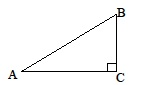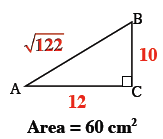Home > A2C > Chapter 1 > Lesson 1.2.4 > Problem1-115

1-115.

The right triangle shown below has a height of $12$ cm, and its area is $60$ square cm. Find $m\measuredangle B$ and the length of the hypotenuse.Use and $A=\frac{1}{2}bh$ solve for $b$.

Use the answer from the previous hint to find the length of $\overline{AB}$.

Try using the Pythagorean Theorem.

Remember that $\text{tan}B=\frac{\text{opp}}{\text{adj}}$.

$m\measuredangle B\approx39.8^\circ\ \ \ \ \sqrt{244}\approx15.62$